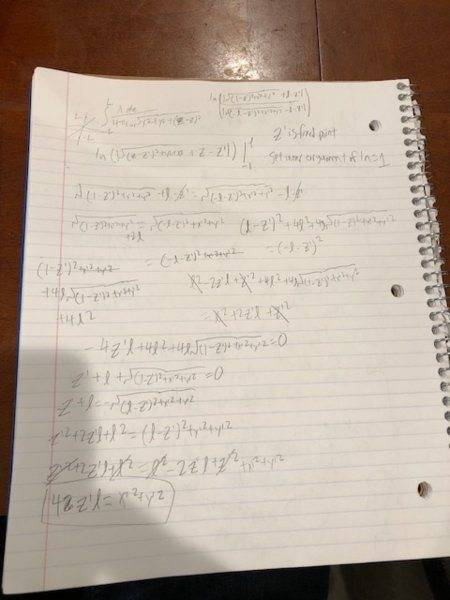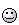# Finding the equipotential surfaces of a finite line of charge

## Homework Statement

Consider a line of charge stretching along the z-axis from -L to +L. Find
the potential everywhere. What are the surfaces of constant potential. (The next question answers the previous question and says its a prolate ellipsoid.

## Homework Equations

I will upload an image of my attempted solution. dV=kdq/r

## The Attempt at a Solution

I got something of the form ax^2 + by^2 = cz#### Attachments

Its supposed to be 4z'l = x'^2 +y'^2

SammyS
Staff Emeritus
Homework Helper
Gold Member

## Homework Statement

Consider a line of charge stretching along the z-axis from -L to +L. Find
the potential everywhere. What are the surfaces of constant potential. (The next question answers the previous question and says its a prolate ellipsoid.

## Homework Equations

I will upload an image of my attempted solution. dV=kdq/r

## The Attempt at a Solution

I got something of the form ax^2 + by^2 = cz
[ ATTACH=full]230780[/ATTACH]
It's extremely difficult to read that image. I did manage to get some idea of what you did.

I wrote essentially what you have for the potential, V, but I write it as the potential at point (x, y, z) and use z' as the integration variable.

Here's the your integral for the potential with the above (minor) modifications in variable names:

## \displaystyle V(x,\,y,\,z) = \frac{\lambda } { 4\pi \varepsilon_0} \int_{-L}^L \frac{ dz' } { \, \sqrt{ x^2 + y^2 + (z' -z)^2 } \,} ##

After following the next few steps I see a problem.

You use a form of the anti-derivative which is in terms of a logarithm. Nothing wrong with that. But if we call that anti-derivative, ##\ \ln(F(z')) \,,\ ## then plugging in the limits of integration gives ##\ \ln(F(L))-\ln(F(-L)) \ \ \rightarrow \ \ \ln \left( \frac{F(L)} {F(-L)} \right)##.

The problem is that then you pick the seemingly convenient choice of solving ## \ F(L) = F(-L) \ since that will make the argument of the logarithm be a constant, namely 1 . The problem with that is this would make the integral equal to zero. That's not possible. The integrand is greater than zero for all values of z' .

As for the answer of being a "prolate ellipsoid", I suppose they mean a prolate spheroid I'm somewhat skeptical of that. Perhaps the equi-potential surfaces approach this shape at a distance sufficiently far from the line charge.

It's extremely difficult to read that image. I did manage to get some idea of what you did.

I wrote essentially what you have for the potential, V, but I write it as the potential at point (x, y, z) and use z' as the integration variable.

Here's the your integral for the potential with the above (minor) modifications in variable names:

## \displaystyle V(x,\,y,\,z) = \frac{\lambda } { 4\pi \varepsilon_0} \int_{-L}^L \frac{ dz' } { \, \sqrt{ x^2 + y^2 + (z' -z)^2 } \,} ##

After following the next few steps I see a problem.

You use a form of the anti-derivative which is in terms of a logarithm. Nothing wrong with that. But if we call that anti-derivative, ##\ \ln(F(z')) \,,\ ## then plugging in the limits of integration gives ##\ \ln(F(L))-\ln(F(-L)) \ \ \rightarrow \ \ \ln \left( \frac{F(L)} {F(-L)} \right)##.

The problem is that then you pick the seemingly convenient choice of solving ## \ F(L) = F(-L) \ since that will make the argument of the logarithm be a constant, namely 1 . The problem with that is this would make the integral equal to zero. That's not possible. The integrand is greater than zero for all values of z' .

As for the answer of being a "prolate ellipsoid", I suppose they mean a prolate spheroid I'm somewhat skeptical of that. Perhaps the equi-potential surfaces approach this shape at a distance sufficiently far from the line charge.
Thanks for responding and for the insight. I was unaware that uploading photos is not the right way to go in the future I will learn latex and use it.

TSny
Homework Helper
Gold Member
As for the answer of being a "prolate ellipsoid", I suppose they mean a prolate spheroid I'm somewhat skeptical of that. Perhaps the equi-potential surfaces approach this shape at a distance sufficiently far from the line charge.
I happen to remember this problem being worked out in book I have. Interestingly, it turns out that the equipotential surfaces are prolate spheroids even when close to the line source.

•SammyS
I happen to remember this problem being worked out in book I have. Interestingly, it turns out that the equipotential surfaces are prolate spheroids even when close to the line source.
Can I ask which book? Our standard text for the class is Technically Griffiths but our professor sometimes likes using Jackson since he himself learned from Jackson. Also I go to Cal if that helps at all.

TSny
Homework Helper
Gold Member
•SammyS
SammyS
Staff Emeritus
Homework Helper
Gold Member
I happen to remember this problem being worked out in book I have. Interestingly, it turns out that the equipotential surfaces are prolate spheroids even when close to the line source.
That amazes me !- especially for locations very close to the line charge relative to L and near the center of the line charge. I would have expected equipotential surfaces there to be virtually cylindrical.

Now, to make a little tweak, to that physical intuition I used to claim and was often proud of.

but ... At my age, maybe no need to bother.TSny
Homework Helper
Gold Member
That amazes me !- especially for locations very close to the line charge relative to L and near the center of the line charge. I would have expected equipotential surfaces there to be virtually cylindrical.
If the charge were spread along a conducting line (needle), then the equipotential surfaces would approach the shape of the needle when you get in close. But, then, the charge density along the needle would be very nonuniform (concentrated near the ends).

Now, to make a little tweak, to that physical intuition I used to claim and was often proud of.

but ... At my age, maybe no need to bother.I'm with you.

By the way, whenever I dip into Becker's book to read about a specific topic, I am usually very pleased with his treatment. The book has an interesting history. See the first paragragh of the forward to the book just before the table of contents at the link https://www.amazon.com/gp/product/0486642909/?tag=pfamazon01-20

The lineage of the book goes all the way back to a popular German text of Foppl (1894), which apparently had some influence on Einstein.
http://einstein-special-relativity.com/august-foppl/

SammyS
Staff Emeritus
Homework Helper
Gold Member
If the charge were spread along a conducting line (needle), then the equipotential surfaces would approach the shape of the needle when you get in close. But, then, the charge density along the needle would be very nonuniform (concentrated near the ends).
Yes, I see. It took me a couple of readings to get what you're saying, but yes, that makes sense.
Considering that I thought the equipotential surfaces would be cylindrical in shape very near the line of charge. If we have such a shape for an equipotential surface, then replacing that surface with a conducting surface of the same shape (and appropriate charge) would produce the the same field at points external to that conductor. Knowing that the majority of charge on such a conductor would migrate to the ends, shows that such an equipotential surface is inconsistent with being due to a uniform distribution on a line segment,

Your explanation did appeal to physical intuition . Thanks!
I'm with you.

By the way, whenever I dip into Becker's book to read about a specific topic, I am usually very pleased with his treatment. The book has an interesting history. See the first paragragh of the forward to the book just before the table of contents at the link https://www.amazon.com/gp/product/0486642909/?tag=pfamazon01-20

The lineage of the book goes all the way back to a popular German text of Foppl (1894), which apparently had some influence on Einstein.
http://einstein-special-relativity.com/august-foppl/
Yes. This book looks very interesting. I may order a copy. (Maybe I'm not too old to see things in a different light.) I would say new light, but the book's roots are even older than I am.